Next: The Fundamental Theorem of Up: Generalization to the n-var Previous: The geometry of n-var

#### Polytope Convexity and Extreme Points

In this paragraph we show that polytopes are convex sets. This property is important for eventually proving the Fundamental Theorem of LP. A set of points S in the n-dim space is convex, if the line segment connecting any two points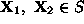, belongs completely in S. According to the previous mathematical definition of line segments, this definition of convexity is mathematically expressed as: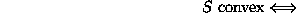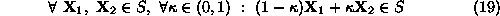Figure 8 depicts the concept.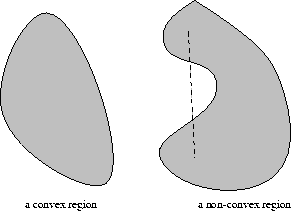Figure 8: Convex sets

To show that a polytope is a convex set, we first establish that the solution space of any linear constraint (i.e., hyperplanes and half-spaces) is a convex set. Since a polytope is the intersection of a number of hyperplanes and half-spaces, the convexity of the latter directly implies the convexity of the polytope (i.e., a line segment belonging to each defining hyperplane and/or half-space will also belong to the polytope).

To establish the convexity of the feasible region of a linear constraint, let's consider the constraint: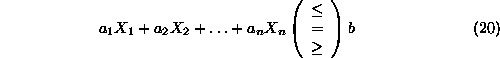and two points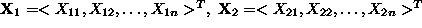satisfying it. Then, for any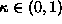, we have: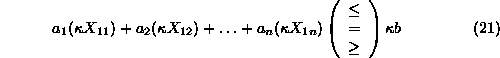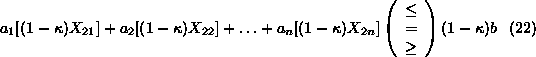Adding Equations 21 and 22, we get: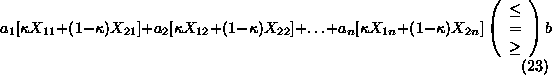i.e., point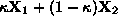belongs in the solution space of the constraint.

A last concept that we must define before the statement of the Fundamental Theorem of LP, is that of the extreme point of a convex set. Given a convex set S, point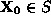is an extreme point, if each line segment that lies completely in S and contains point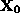, hasas an end point of the line segment. Mathematically,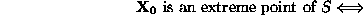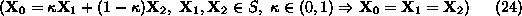UAL Data
Fri Jun 20 15:03:05 CDT 1997